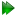Subsections

Double rotor equations

The double rotor model consists of two standard rotations, the first about the x-axis and the second about the y-axis. Hence the frame order matrix is simply the integration over both torsion angles of the Kronecker product of the product of the Rx and Ry rotation matrices, divided by the surface area normalisation factor.

Figure 16.27: The double rotor model simulated and calculated in-frame Daeg(1) frame order matrix elements. In these plots, θX corresponds to the torsion half-angle σmax, 1 and θY to the torsion half-angle σmax, 2. When the half-angle is not varied, the angle is fixed to either σmax, 1 = π/4 or σmax, 2 = 3π/8. Frame order matrix values have been calculated every 10 degrees.Figure 16.28: The double rotor model simulated and calculated in-frame Daeg(2) frame order matrix elements. In these plots, θX corresponds to the torsion half-angle σmax, 1 and θY to the torsion half-angle σmax, 2. When the half-angle is not varied, the angle is fixed to either σmax, 1 = π/4 or σmax, 2 = 3π/8. Frame order matrix values have been calculated every 10 degrees.Figure 16.29: The double rotor model simulated and calculated out-of-frame Daeg(1) frame order matrix elements. In these plots, θX corresponds to the torsion half-angle σmax, 1 and θY to the torsion half-angle σmax, 2. When the half-angle is not varied, the angle is fixed to either σmax, 1 = π/4 or σmax, 2 = 3π/8. Frame order matrix values have been calculated every 10 degrees.Figure 16.30: The double rotor model simulated and calculated out-of-frame Daeg(2) frame order matrix elements. In these plots, θX corresponds to the torsion half-angle σmax, 1 and θY to the torsion half-angle σmax, 2. When the half-angle is not varied, the angle is fixed to either σmax, 1 = π/4 or σmax, 2 = 3π/8. Frame order matrix values have been calculated every 10 degrees.Double rotor rotation matrices

The individual rotations areThe full rotation is thenDouble rotor frame order matrix

The frame order matrix is

 Daeg(n) =R(σ1, σ2)⊗n dSdS, (16.68) =R(σ1, σ2)⊗n dσmax, 1 dσmax, 2dS. (16.69)

The surface normalisation factor isDouble rotor 1 degree frame order

The un-normalised 1 degress frame order matrix with tensor rank-2 isAfter normalisation, the full frame order matrix is

 Daeg(1) =. (16.72)

Double rotor 2 degree frame order

The 2 degree frame order matrix with tensor rank-4 consists of the following elements, using Kronecker product double indices from 0 to 8Double rotor frame order matrix simulation and calculation

The frame order matrix element simulation script from Section 16.2, pagewas used to compare the implementation of equations 16.72 and 16.73 above. Frame order matrix Daeg(1) and Daeg(2) values were both simulated and calculated, both within and out of the motional eigenframe. The in-frame Daeg(1) values are shown in figure 16.27 and Daeg(2) in figure 16.28. The out-of-frame Daeg(1) values are shown in figure 16.29 and Daeg(2) in figure 16.30.

The relax user manual (PDF), created 2020-08-26.